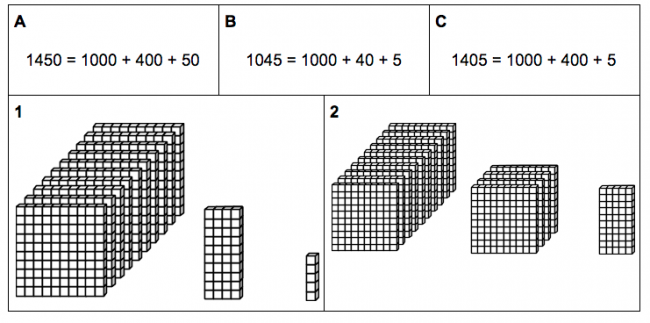# Place Value to 1000 #1

Preview:This task allows students to see the scale of the quantities for thousands, hundreds, tens, and ones in place value. Students match one of the three equations given to one of the visuals given, then create the missing visual for the third equation.

Connecting Representations
Big Idea:
Grade 4: Development of computational fluency and multiplicative thinking requires analysis of patterns and relations in multiplication and division.
Content:
Numbers to 10 000 can be arranged and recognized: comparing and ordering numbers estimating large quantities Place value: 1000s, 100s, 10s, and 1s understanding the relationship between digit places and their value, to 10 000
Curricular Competency:
Reasoning and analyzing: Develop mental math strategies and abilities to make sense of quantities
Understanding and solving: Develop, demonstrate, and apply mathematical understanding through play, inquiry, and problem solving
Understanding and solving: Visualize to explore mathematical concepts
Understanding and solving: Develop and use multiple strategies to engage in problem solving
Communicating and representing: Communicate mathematical thinking in many ways
Communicating and representing: Use mathematical vocabulary and language to contribute to mathematical discussions
Communicating and representing: Explain and justify mathematical ideas and decisions
Communicating and representing: Represent mathematical ideas in concrete, pictorial, and symbolic forms
Connecting and reflecting: Reflect on mathematical thinking